Newbie

# 9. If tan θ = a/b, find the value of (cos θ + sin θ)/ (cos θ – sin θ)

• 0

Explain the method used.

Class 10th, Rd sharma Trigonometric identities.

Share

1. Solution: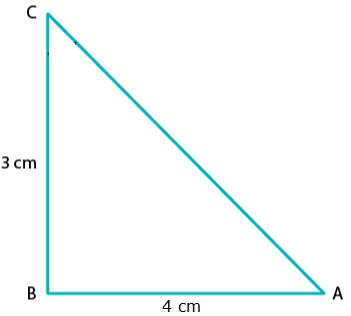Given,

3cot A = 4

⇒ cot A = 4/3

By definition,

tan A = 1/ Cot A = 1/ (4/3)

⇒ tan A = 3/4

Thus,

Base side adjacent to ∠A = 4

Perpendicular side opposite to ∠A = 3

In ΔABC, Hypotenuse is unknown.

Thus, by applying Pythagoras theorem in ΔABC,

We get

AC= AB2 + BC2

AC2 = 42 + 32

AC2 = 16 + 9

AC2 = 25

AC = √25

AC = 5

Hence, hypotenuse = 5

Now, we can find that

sin A = opposite side to ∠A/ Hypotenuse = 3/5

And,

cos A = adjacent side to ∠A/ Hypotenuse = 4/5

Taking the LHS,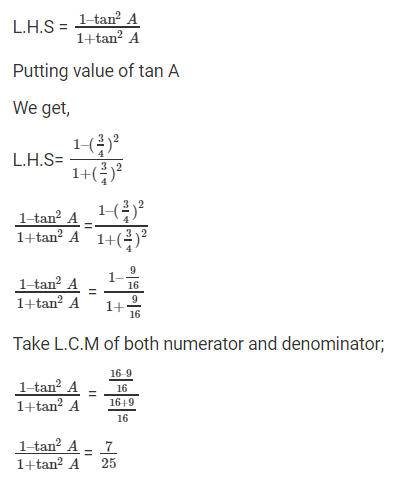Thus, LHS = 7/25

Now, taking RHS,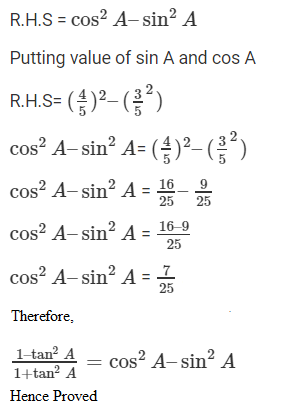• 0
2. Solution:

Given,

tan θ = a/b

And we know by definition that

tan θ = opposite side/ adjacent side

Thus, by comparison,

Opposite side = a and adjacent side = b

To find the hypotenuse, we know that by Pythagoras theorem that

Hypotenuse2 = opposite side2 + adjacent side2

⇒ Hypotenuse = √(a2 + b2)

So, by definition

sin θ = opposite side/ Hypotenuse

sin θ = a/ √(a2 + b2)

And,

cos θ = adjacent side/ Hypotenuse

cos θ = b/ √(a2 + b2)

Now,

After substituting for cos θ and sin θ, we have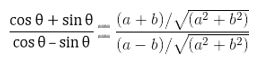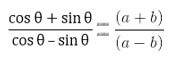Hence, proved.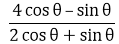• 0